# Sin, Cos and Tan Graphs

GCSELevel 6-7AQACambridge iGCSEEdexcelEdexcel iGCSEOCRWJEC

## Sin, Cos & Tan Graphs

Sine, cosine and tangent graphs are specific graphs you need to be able to identify, understand and draw.

Specifically, the graphs of

$y=\textcolor{blue}{\sin} x,\,\,\,\,y=\textcolor{limegreen}{\cos} x,\,\,\,\,\text{and}\,\,\,\,y=\textcolor{red}{\tan} x$.

You may be asked to draw graphs for any values of $x\degree$, these graphs are periodic, which means that after a certain point, the graph follows a pattern and repeats itself over and over.

Make sure you are happy with the following topics before moving on:

Level 6-7GCSEAQAEdexcelOCRWJECCambridge iGCSEEdexcel iGCSE

## Sine Graphs

The graph

$y=\sin x$ between $0\degree$ and $360\degree$

is the graph shown below.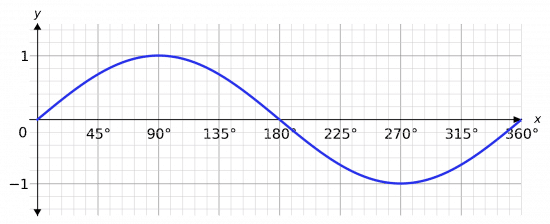The key features of this graph you need to remember:

• The peak is $\mathbf{1}$ and occurs at $\mathbf{90}\degree$,
• The minimum is $\mathbf{-1}$ and occurs at $\mathbf{270}\degree$,
• The graph crosses the axis at $\mathbf{0}\degree, \mathbf{180}\degree,$ and $\mathbf{360}\degree$.

As mentioned, this is one period, which means that past $360\degree$ and before $0\degree$, it repeats this exact same shape which lasts for $360\degree$.

This is shown below.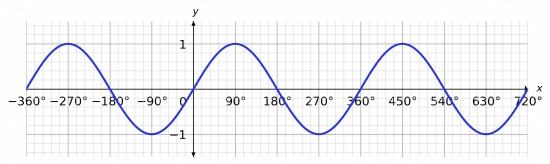Level 6-7GCSEAQAEdexcelOCRWJECCambridge iGCSEEdexcel iGCSE

## Cosine Graphs

The graph

$y=\cos x$ between $0\degree$ and $360\degree$

is shown below.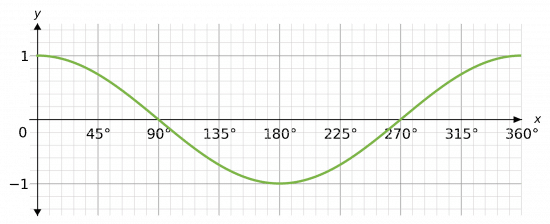The key features of this graph you need to remember:

• The peak is $\mathbf{1}$ and occurs at $\mathbf{0}\degree$ and $\mathbf{360}\degree$,
• The minimum is $\mathbf{-1}$ and occurs at $\mathbf{180}\degree$,
• The graph crosses the axis at $\mathbf{90}\degree$ and $\mathbf{270}\degree$.

As with the sine graph, this portion is one period of the graph, so it is repeated for all the values before $0\degree$ and past $360\degree.$

If we repeat this period a few times, we will see that the shape is exactly the same as the sine graph.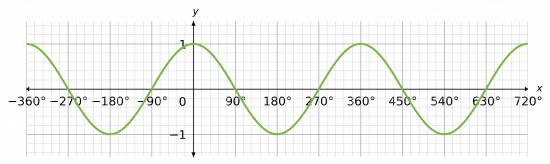NOTE: If, at any point, you can remember the general shape of these graphs but can’t remember which graph is which, you can recall/calculate the values of $\sin$ and $\cos$ at zero, and then extend the pattern from there onward.

Level 6-7GCSEAQAEdexcelOCRWJECCambridge iGCSEEdexcel iGCSE

## Tangent Graphs

The graph

$y=\tan x$ between $-90\degree$ and $90\degree$

is very different, it looks like the graph shown below.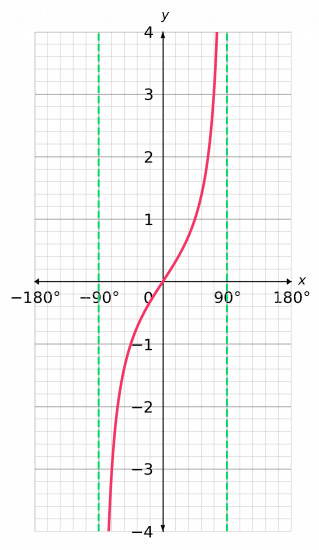• It crosses the axes once at the origin,
• The graph gets very big as the angle gets close to $90\degree$, and similarly gets very small as the angle gets close to $-90\degree$,
• The dotted lines on this graph are asymptotes – lines which the function gets closer and closer to but never quite touches.

As with the previous graphs, this part only represents one period. However, this period repeats every $180\degree$, unlike the previous graphs that are repeated every $360\degree$.

Note: as the graph repeats, so do the asymptotes.

The result of repeating the shape a few times is shown below.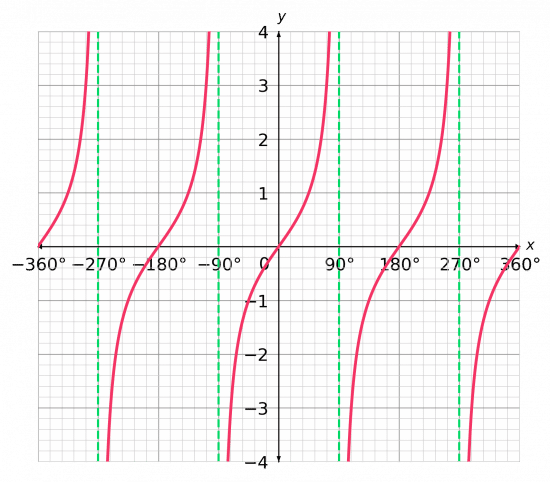If anything, this graph is slightly simpler than the previous two, because it only crosses the axis once every $180\degree$.

Level 6-7GCSEAQAEdexcelOCRWJECCambridge iGCSEEdexcel iGCSE

## Sin, Cos and Tan Graphs Example Questions

If you can’t remember their shapes, check a few points. So, we have that

$\cos(0)=1,\,\,\text{ and }\,\,\cos(90)=0$

Which is enough to start of the pattern of the $\cos$ graph. Similarly, we have

$\sin(0)=0,\,\,\text{ and }\,\,\sin(90)=1$

Which is enough to start the pattern of the $\sin$ graph. If you aren’t sure, just try more values. The resulting graph looks like: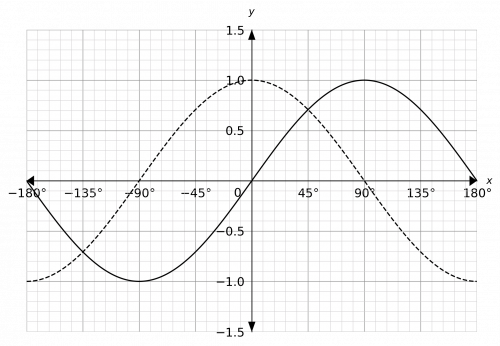The solid black line represents the $\sin$ graph and the dotted line represents the $\cos$ graph.Gold Standard Education

The $\tan$ graph has an asymptote at $90\degree$, and then again every $180\degree$ before and after that. Furthermore, we have that $\tan(0)=0$ and it gets bigger as it gets close to $90\degree$. This enough to draw the graph. The result looks like: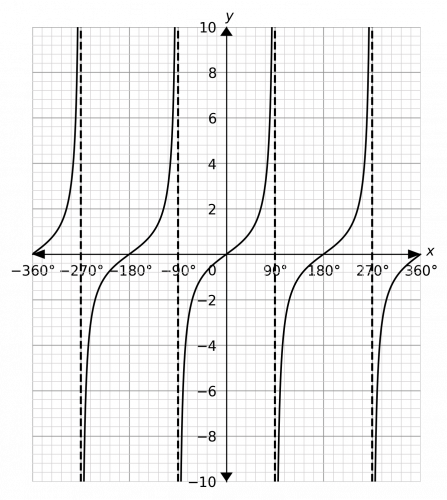Gold Standard Education

This is a transformation of the form $y=-f(x)$, which corresponds to a reflection in the $x$ axis. In doing this, it would be helpful for you to draw a normal $\cos$ graph, draw the reflection, and then rub out the first one.

To draw the $\cos$ graph, consider that

$\cos(0)=1\,\,\text{ and }\,\,\cos(90)=0$

This is enough to continue the pattern to $360\degree$. The resulting graph should look like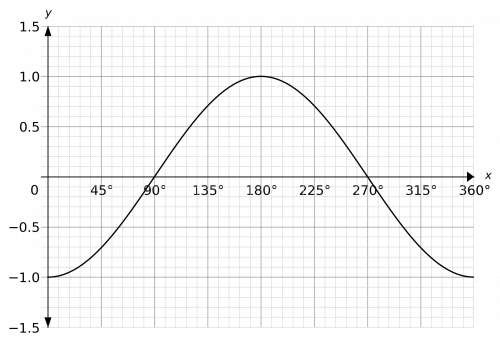Gold Standard Education

Here we will plot $y=\sin(x)$ as a dotted line and $y=2\sin(x)$ as a solid line. The resulting graph should look like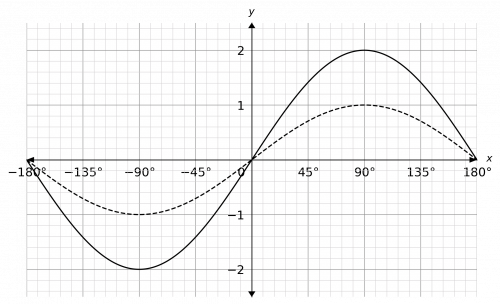Gold Standard Education

## Sin, Cos and Tan Graphs Worksheet and Example Questions

### (NEW) Sin, Cos and Tan graphs Exam Style Questions - MME

Level 6-7GCSENewOfficial MME

## Sin, Cos and Tan Graphs Drill Questions

### Sine Cos and Tan Graphs

Level 6-7GCSEGold Standard Education

## Related Topics

#### Coordinates

Level 4-5Level 6-7KS3

Level 1-3KS3

#### Functions

Level 4-5Level 6-7